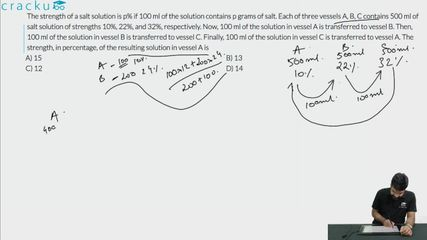Question 80

# The strength of a salt solution is p% if 100 ml of the solution contains p grams of salt. Each of three vessels A, B, C contains 500 ml of salt solution of strengths 10%, 22%, and 32%, respectively. Now, 100 ml of the solution in vessel A is transferred to vessel B. Then, 100 ml of the solution in vessel B is transferred to vessel C. Finally, 100 ml of the solution in vessel C is transferred to vessel A. The strength, in percentage, of the resulting solution in vessel A is

Solution

Each of three vessels A, B, C contains 500 ml of salt solution of strengths 10%, 22%, and 32%, respectively.

The amount of salt in vessels A, B, C = 50 ml, 110 ml, 160 ml respectively.

The amount of water in vessels A, B, C = 450 ml, 390 ml, 340 ml respectively.

In 100 ml solution in vessel A, there will be 10ml of salt and 90 ml of water

Now, 100 ml of the solution in vessel A is transferred to vessel B. Then, 100 ml of the solution in vessel B is transferred to vessel C. Finally, 100 ml of the solution in vessel C is transferred to vessel A

i.e after the first transfer, the amount of salt in vessels A, B, C = 40, 120, 160 ml respectively.

after the second transfer, the amount of salt in vessels A, B, C =40, 100, 180 ml respectively.

After the third transfer, the amount of salt in vessels A, B, C = 70, 100, 150 respectively.

Each transfer can be captured through the following table.

Percentage of salt in vessel A =$$\ \frac{\ 70}{500}\times\ 100$$

=14%

### View Video Solution